# "Draw a graph depicting a skydivers speed against time when jumping from a plane, until he deploys his parachute, explaining the logic of your answer through the forces applicable to the body."

Because we’re interested in seeing how the skydiver’s speed is changing with time, a velocity-time graph will be most relevant to us: not only does it have all the quantities we need, but it also has something important hidden within its gradient - the acceleration of the skydiver! That will give us interesting insight about the resultant force acting on the skydiver is changing with time.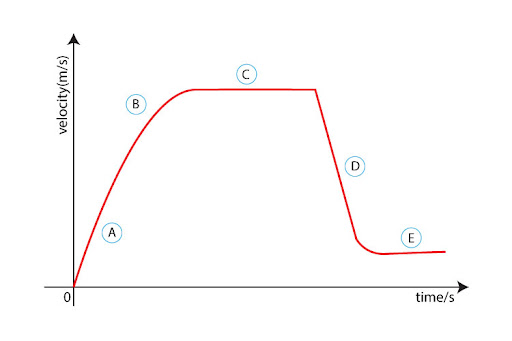Now let us dive into each section of this rollercoaster-like graph:

A: Initial Acceleration:
At the very start, right when the skydiver jumps off their plane, the dominant force acting is weight, acting vertically downwards. It is only after a short interval of time, when the skydiver picks up some speed, that drag force starts acting vertically upwards. With increasing speed, this drag force keeps on increasing until…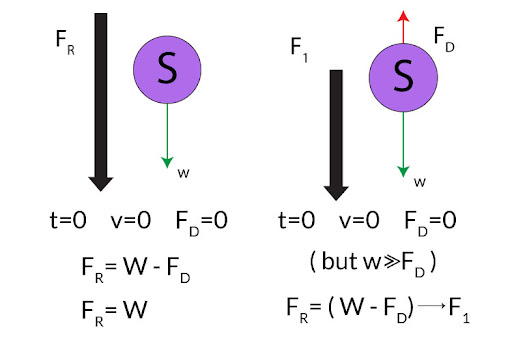B: Slowing Down:
… This is where the drag force is gradually catching up on weight, getting larger and larger. Ultimately, this causes the overall resultant force - and acceleration - to get smaller and smaller. This decreasing acceleration is what’s causing the graph to curl somewhat inwards, showing that the skydiver’s speed is increasing slowly with respect to time.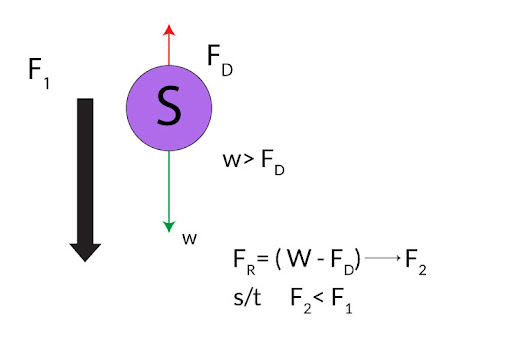C: Terminal Velocity
Now we have reached the point when drag force becomes exactly equal to the skydiver’s weight. Resultant force is now zero - and since F = ma, and a = F/m, therefore acceleration is also zero. That means the skydiver has now attained a maximum constant speed we call terminal velocity. Notice how the horizontal line shows zero gradient - meaning zero acceleration!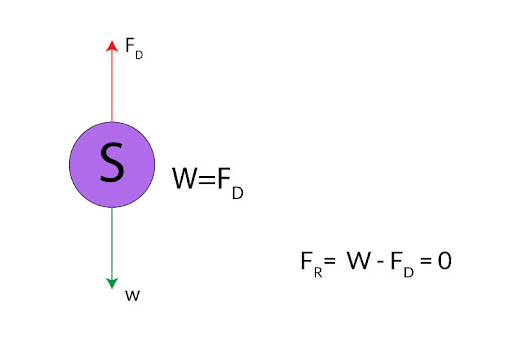D: Parachute Open!

Finally, the skydiver unleashes their parachute - and their speed drops almost instantaneously! That’s because the open parachute offers a large surface area exposed to the air. Because of more exposure, the amount of air resistance resisting the skydiver’s motion will drastically increase. We can prove that through the equation of drag force as well: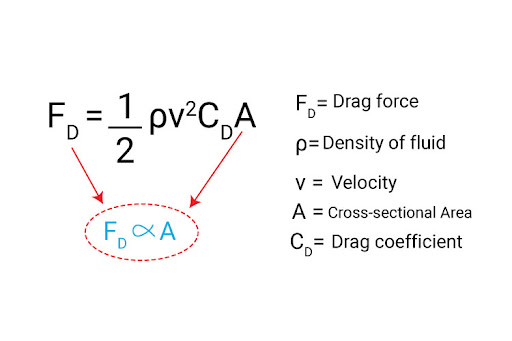So now we see that indeed, drag force does increase dramatically - so much so that it ends up exceeding weight! What does that do to the resultant force? Well, we now see that the resultant force is not only non-zero, but it is also directed upwards. A negative resultant force gives rise to deceleration, hence why velocity drops to a smaller value.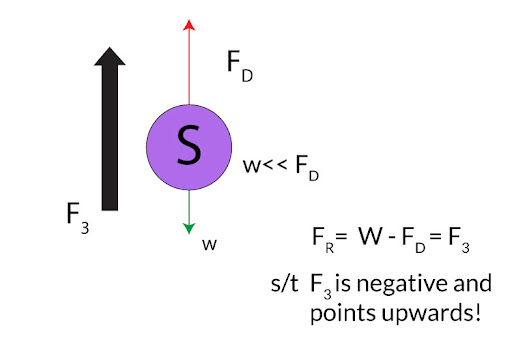Of course, the velocity doesn’t go on to decrease further and further - do remember that as the velocity drops, so will the drag force! And because the decrease in velocity is more drastic than the increase in air resistance, it eventually overrides such that drag force starts decreasing once more to equalize with weight. That’s when acceleration reduces to zero again, leading to a new lower terminal velocity (and that’s why you see a lower horizontal line!)